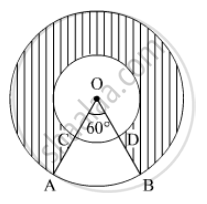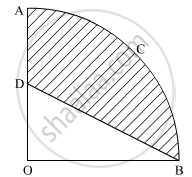# Important Questions for CBSE Class 10 - CBSE - Mathematics

Subjects
Topics
Subjects
Popular subjects
Topics
Mathematics
< prev 1 to 20 of 1144 next >

Prove that the lengths of the tangents drawn from an external point to a circle are equal.

Appears in 20 question papers
Chapter: [3.01] Circles
Concept: Number of Tangents from a Point on a Circle

Prove that the tangent at any point of a circle is perpendicular to the radius through the point of contact.

Appears in 14 question papers
Chapter: [3.01] Circles
Concept: Tangent to a Circle

Prove that a parallelogram circumscribing a circle is a rhombus.

Appears in 8 question papers
Chapter: [3.01] Circles
Concept: Number of Tangents from a Point on a Circle

A bucket open at the top is in the form of a frustum of a cone with a capacity of 12308.8 cm3. The radii of the top and bottom circular ends are 20 cm and 12 cm, respectively. Find the height of the bucket and the area of metal sheet used in making the bucket. (use π = 3.14)

Appears in 6 question papers
Chapter: [4.01] Heights and Distances
Concept: Heights and Distances

Prove that opposite sides of a quadrilateral circumscribing a circle subtend supplementary angles at the centre of the circle.

Appears in 5 question papers
Chapter: [3.01] Circles
Concept: Number of Tangents from a Point on a Circle

Prove that the tangents drawn at the ends of a diameter of a circle are parallel.

Appears in 5 question papers
Chapter: [3.01] Circles
Concept: Number of Tangents from a Point on a Circle

In Figure , two concentric circles with centre O, have radii 21cm and 42 cm. If ∠ AOB = 60°, find the area of the shaded region. [use π=22/7]Appears in 5 question papers
Chapter: [7.01] Areas Related to Circles
Concept: Circumference of a Circle

In Figure , two concentric circles with centre O, have radii 21cm and 42 cm. If ∠ AOB = 60°, find the area of the shaded region. [use π=22/7]Appears in 5 question papers
Chapter: [7.01] Areas Related to Circles
Concept: Circumference of a Circle

In an AP of 50 terms, the sum of first 10 terms is 210 and the sum of its last 15 terms is 2565. Find the A.P.

Appears in 4 question papers
Chapter: [2.02] Arithmetic Progressions
Concept: Arithmetic Progression

Solve the following quadratic equation for x: 4x^2 + 4bx – (a^2 – b^2) = 0

Appears in 4 question papers
Concept: Solutions of Quadratic Equations by Completing the Square

A motorboat whose speed is 18 km/hr in still water takes 1 hr more to go 24 km upstream than to return downstream to the same spot. Find the speed of the stream.

Appears in 4 question papers
Chapter: [2.04] Polynomials
Concept: Concept of Polynomials

A motorboat whose speed is 18 km/hr in still water takes 1 hr more to go 24 km upstream than to return downstream to the same spot. Find the speed of the stream.

Appears in 4 question papers
Chapter: [2.04] Polynomials
Concept: Concept of Polynomials

Construct a triangle ABC with sides BC = 7 cm, ∠B = 45° and ∠A = 105°. Then construct a triangle whose sides are 3/4 times the corresponding sides of ∆ABC.

Appears in 4 question papers
Chapter: [3.02] Triangles
Concept: Application of Pythagoras Theorem in Acute Angle and Obtuse Angle

In the given figure, OACB is a quadrant of a circle with centre O and radius 3.5 cm. If OD = 2 cm, find the area of the shaded region.Appears in 4 question papers
Chapter: [7.01] Areas Related to Circles
Concept: Areas of Sector and Segment of a Circle

Water is flowing at the rate of 2.52 km/h through a cylindrical pipe into a cylindrical tank, the radius of whose base is 40 cm. If the increase in the level of water in the tank, in half an hour is 3.15 m, find the internal diameter of the pipe.

Appears in 4 question papers
Chapter: [7.02] Surface Areas and Volumes
Concept: Concept of Surface Area, Volume, and Capacity

Water is flowing at the rate of 2.52 km/h through a cylindrical pipe into a cylindrical tank, the radius of whose base is 40 cm. If the increase in the level of water in the tank, in half an hour is 3.15 m, find the internal diameter of the pipe.

Appears in 4 question papers
Chapter: [7.02] Surface Areas and Volumes
Concept: Concept of Surface Area, Volume, and Capacity

What is the HCF of the smallest prime number and the smallest composite number?

Appears in 3 question papers
Chapter: [1.01] Real Numbers
Concept: Fundamental Theorem of Arithmetic

Given that sqrt2 is irrational prove that (5 + 3sqrt2)  is an irrational number

Appears in 3 question papers
Chapter: [1.01] Real Numbers
Concept: Concept of Irrational Numbers

Find HCF and LCM of 404 and 96 and verify that HCF × LCM = Product of the two given numbers.

Appears in 3 question papers
Chapter: [1.01] Real Numbers
Concept: Fundamental Theorem of Arithmetic

A shopkeeper buys some books for Rs 80. If he had bought 4 more books for the same amount, each book would have cost Rs 1 less. Find the number of books he bought.

Appears in 3 question papers
Chapter: [1.01] Real Numbers
Concept: Real Numbers Examples and Solutions
< prev 1 to 20 of 1144 next >
Important Questions for CBSE Class 10 CBSE Mathematics. You can further filter Important Questions by subjects and topics. Chapter wise important Questions for Class 10 CBSE. it gets easy to find all Class 10 important questions with answers in a single place for students. Saving time and can then focus on their studies and practice. Important questions for Class 10 chapter wise with solutions

Class 10 Mathematics can be very challenging and complicated. But with the right ideas and support, you will be able to make it work. That’s why we have the class 10 Mathematics important questions with answers pdf ready for you. All you need is to acquire the PDF with all the content and then start preparing for the exam. It really helps and it can bring in front an amazing experience every time if you're tackling it at the highest possible level.

We make sure that you have access to the important questions for Class 10 Mathematics CBSE. This way you can be fully prepared for any specific question without a problem. There are a plethora of different questions that you need to prepare. And that's why we are covering the most important ones. They are the questions that will end up being more and more interesting and the results themselves can be staggering every time thanks to that. The quality itself will be quite amazing every time, and you can check the important questions for Class 10 Mathematics CBSE whenever you see fit.

The important questions for Class 10 Mathematics 2021 we provide on this page are very accurate and to the point. You will have all the information already prepared and that will make it a lot simpler to ace the exam. Plus, you get to browse through these important questions for Class 10 Mathematics 2021 and really see what works, what you need to adapt or adjust and what still needs some adjustment in the long run. It's all a matter of figuring out these things and once you do that it will be a very good experience.

All you have to do is to browse the important questions for upcoming CBSE Class 10 2021 on our website. Once you do, you will know what needs to be handled, what approach works for you and what you need to do better. That will certainly be an incredible approach and the results themselves can be nothing short of staggering every time. We encourage you to browse all these amazing questions and solutions multiple times and master them. Once you do that the best way you can, you will have a much higher chance of passing the exam, and that's what really matters the most!